SSC CGL Previous Year Questions: Trigonometry - 4

# SSC CGL Previous Year Questions: Trigonometry - 4 - SSC CGL

Test Description

## 40 Questions MCQ Test SSC CGL (Tier - 1) - Previous Year Papers (Topic Wise) - SSC CGL Previous Year Questions: Trigonometry - 4

SSC CGL Previous Year Questions: Trigonometry - 4 for SSC CGL 2023 is part of SSC CGL (Tier - 1) - Previous Year Papers (Topic Wise) preparation. The SSC CGL Previous Year Questions: Trigonometry - 4 questions and answers have been prepared according to the SSC CGL exam syllabus.The SSC CGL Previous Year Questions: Trigonometry - 4 MCQs are made for SSC CGL 2023 Exam. Find important definitions, questions, notes, meanings, examples, exercises, MCQs and online tests for SSC CGL Previous Year Questions: Trigonometry - 4 below.
Solutions of SSC CGL Previous Year Questions: Trigonometry - 4 questions in English are available as part of our SSC CGL (Tier - 1) - Previous Year Papers (Topic Wise) for SSC CGL & SSC CGL Previous Year Questions: Trigonometry - 4 solutions in Hindi for SSC CGL (Tier - 1) - Previous Year Papers (Topic Wise) course. Download more important topics, notes, lectures and mock test series for SSC CGL Exam by signing up for free. Attempt SSC CGL Previous Year Questions: Trigonometry - 4 | 40 questions in 40 minutes | Mock test for SSC CGL preparation | Free important questions MCQ to study SSC CGL (Tier - 1) - Previous Year Papers (Topic Wise) for SSC CGL Exam | Download free PDF with solutions
 1 Crore+ students have signed up on EduRev. Have you?
SSC CGL Previous Year Questions: Trigonometry - 4 - Question 1

### (3π/5) radians is equal to   (SSC CGL 1st Sit. 2013)

Detailed Solution for SSC CGL Previous Year Questions: Trigonometry - 4 - Question 1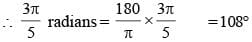SSC CGL Previous Year Questions: Trigonometry - 4 - Question 2

### Evaluate: 3 cos 80° cosec 10° + 2 cos 59° cosec 31°    (SSC CGL 1st Sit. 2013)

Detailed Solution for SSC CGL Previous Year Questions: Trigonometry - 4 - Question 2

3 cos 80°. cosec 10° + 2 cos 59°. cosec 31°
= 3 cos (90° – 10°). cosec 10° + 2 cos (90° – 31°). cosec 31°
= 3 sin 10°. cosec 10° + 2 sin 31°. cosec 31° = 3 + 2 = 5
[∵ cos (90° – θ) = sinθ; sinθ.cosecθ = 1]

SSC CGL Previous Year Questions: Trigonometry - 4 - Question 3

### If the angles of elevation of a balloon from two consecutive kilometre–stones along a road are 30° and 60° respectively, then the height of the balloon above the ground will be    (SSC CGL 1st Sit. 2013)

Detailed Solution for SSC CGL Previous Year Questions: Trigonometry - 4 - Question 3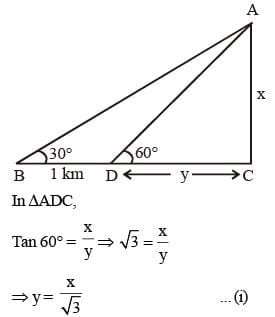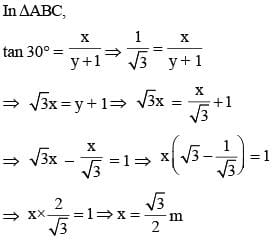SSC CGL Previous Year Questions: Trigonometry - 4 - Question 4

From 125 metre high towers, the angle of depression of a car is 45°. Then how far the car is from the tower?   (SSC CGL 1st Sit. 2013)

Detailed Solution for SSC CGL Previous Year Questions: Trigonometry - 4 - Question 4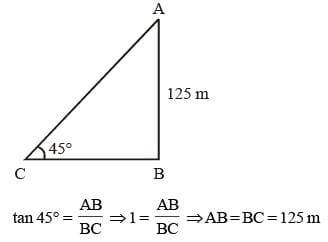SSC CGL Previous Year Questions: Trigonometry - 4 - Question 5

If tan α + cot α = 2, then the value of tan 7α + cot7α is    (SSC CGL 1st Sit. 2013)

Detailed Solution for SSC CGL Previous Year Questions: Trigonometry - 4 - Question 5

tan α + cot α = 2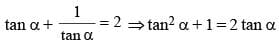⇒ tan2 α – 2 tan α + 1 = 0
⇒ tan2 α – tan α – tan α + 1 = 0
⇒ tan α (tan α – 1) – 1 (tan α – 1) = 0 (tan α – 1) (tan α – 1) = 0
∴ tan α = 1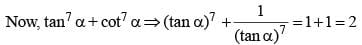SSC CGL Previous Year Questions: Trigonometry - 4 - Question 6

If x = a cos θ – b sin θ, y = b cos θ + a sin θ, then find the value of x2 + y2.    (SSC CGL 1st Sit. 2013)

Detailed Solution for SSC CGL Previous Year Questions: Trigonometry - 4 - Question 6

x = a cos θ – b sin θ
y = b cos θ + a sin θ
x2 + y2 = (a cos θ – b sin θ)2 + (b cos θ + a sin θ)2 = a2 cos2 θ + b2 sin2 θ + a2 sin2 θ + b2 cos2 θ
= a2 + b2

SSC CGL Previous Year Questions: Trigonometry - 4 - Question 7

In a triangle, the angles are in the ratio 2 : 5 : 3. What is the value of the least angle in the radian?    (SSC CGL 1st Sit. 2013)

Detailed Solution for SSC CGL Previous Year Questions: Trigonometry - 4 - Question 7

Let angles are 2x, 5x and 3x.
2x + 5x + 3x = 180º (sum of interior angle of triangles is 180º)
10x = 18º
x = 18º
∴ Least angle in degree = 2x = 2 × 18 = 36º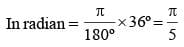SSC CGL Previous Year Questions: Trigonometry - 4 - Question 8

The value of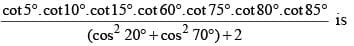(SSC CGL 1st Sit. 2013)

Detailed Solution for SSC CGL Previous Year Questions: Trigonometry - 4 - Question 8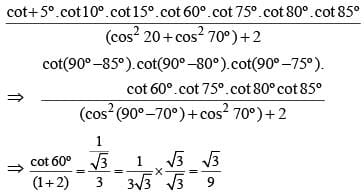SSC CGL Previous Year Questions: Trigonometry - 4 - Question 9

The value of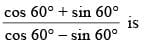(SSC CGL 1st Sit. 2013)

Detailed Solution for SSC CGL Previous Year Questions: Trigonometry - 4 - Question 9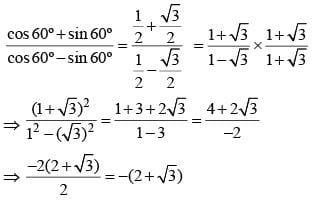SSC CGL Previous Year Questions: Trigonometry - 4 - Question 10

The value of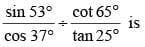(SSC CGL 1st Sit. 2013)

Detailed Solution for SSC CGL Previous Year Questions: Trigonometry - 4 - Question 10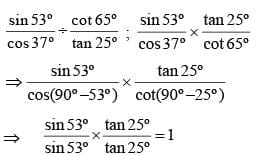[∵ cos (90º – θ) = sin θ and cot (90º – θ) = tan θ]

SSC CGL Previous Year Questions: Trigonometry - 4 - Question 11

If cos4θ – sin4θ = 2/3, then the value of 1 – 2 sin2θ is    (SSC CGL 1st Sit. 2013)

Detailed Solution for SSC CGL Previous Year Questions: Trigonometry - 4 - Question 11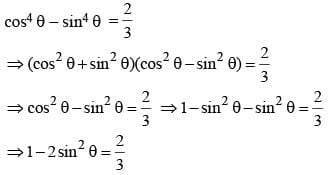SSC CGL Previous Year Questions: Trigonometry - 4 - Question 12

If cosθ + sinθ = √2 cos θ, then cosθ – sinθ is    (SSC CGL 1st Sit. 2013)

Detailed Solution for SSC CGL Previous Year Questions: Trigonometry - 4 - Question 12

cosθ + sinθ = √2 cos θ
On squaring both sides,
cos2θ + sin2θ + 2cos θ. sin θ = 2 cos2 θ
⇒ cos2θ – sin2θ = 2cosθ. cos θ
⇒ (cos θ + sin θ) (cos θ – sin θ) = 2 sin θ. cos θ
⇒ 2 cos θ (cosθ – sinθ) = 2sin θ. cos θ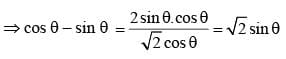SSC CGL Previous Year Questions: Trigonometry - 4 - Question 13

The value of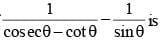(SSC CGL 1st Sit. 2013)

Detailed Solution for SSC CGL Previous Year Questions: Trigonometry - 4 - Question 13

Expression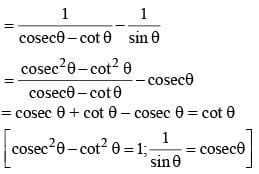SSC CGL Previous Year Questions: Trigonometry - 4 - Question 14

If tan α = n tan β, and sin α = m sin β, then cos2 α is    (SSC CGL 1st Sit. 2013)

Detailed Solution for SSC CGL Previous Year Questions: Trigonometry - 4 - Question 14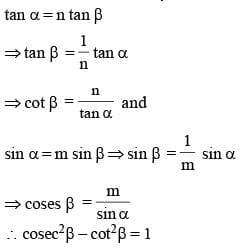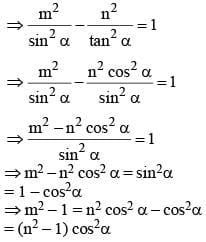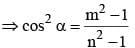SSC CGL Previous Year Questions: Trigonometry - 4 - Question 15

The tops of two poles of height 24 m and 36 m are connected by a wire. If the wire makes an angle of 60° with the horizontal, then the length of the wire is    (SSC CGL 1st Sit. 2013)

Detailed Solution for SSC CGL Previous Year Questions: Trigonometry - 4 - Question 15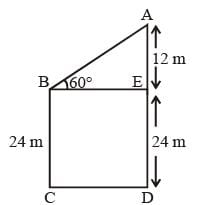BC = ED = 24 m
∴ AE = AD – ED = 36 m  – 24 m = 12 m
In ΔABE,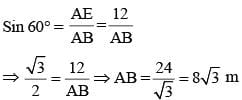SSC CGL Previous Year Questions: Trigonometry - 4 - Question 16

The value of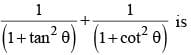(SSC CGL 1st Sit. 2013)

Detailed Solution for SSC CGL Previous Year Questions: Trigonometry - 4 - Question 16

Expression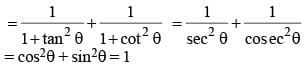SSC CGL Previous Year Questions: Trigonometry - 4 - Question 17

If tan θ = 3/4 and θ is acute, then cosec θ   (SSC CGL 1st Sit. 2013)

Detailed Solution for SSC CGL Previous Year Questions: Trigonometry - 4 - Question 17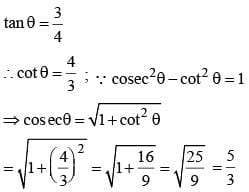SSC CGL Previous Year Questions: Trigonometry - 4 - Question 18

One of the four an gles of a rhombus is 60°. If the length of each side of the rhombus is, 8 cm, then the length of the longer diagonal is    (SSC CGL 2nd Sit. 2013)

Detailed Solution for SSC CGL Previous Year Questions: Trigonometry - 4 - Question 18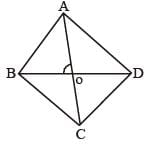∴ ∠BAO = 30°
∠ABO = 60°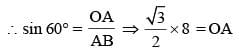∴ OA = 43
∴ AC = 83 metre

SSC CGL Previous Year Questions: Trigonometry - 4 - Question 19

If (1 + sinα) (1 + sinβ) (1 + sinγ) = (1 – sinα) (1 – sinβ) (1 – sinγ), then each side is equal to   (SSC CGL 2nd Sit. 2013)

Detailed Solution for SSC CGL Previous Year Questions: Trigonometry - 4 - Question 19

(1 + sin α) (1 + sin β) (1 + sin γ) = (1 – sin α)  (1 – sin β) (1 – sin γ) = x
∴ x.x = (1 + sin α) (1– sin α) (1 + sin β)
(1 – sin β) (1 + sin γ) (1 – sin γ)
x2 = (1 – sin2 α) (1 – sin2 β) (1 – sin2 γ)
x2 = cos2 α. cos2β.cos2 γ
⇒ x = ± cos α. cos β . cos γ

SSC CGL Previous Year Questions: Trigonometry - 4 - Question 20

The value of 152 (sin 30° + 2 cos2 45° + 3 sin 30° + 4 cos2 45° +...+17 sin 30° + 18 cos45°) is    (SSC CGL 2nd Sit. 2013)

Detailed Solution for SSC CGL Previous Year Questions: Trigonometry - 4 - Question 20

152 (sin 30° + 2 cos2 45° + 3 sin 30° + 4 cos2 45° +...+17 sin 30° + 18 cos45°)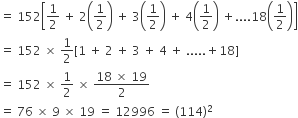Thus, Required number is a perfect square of an integer.

SSC CGL Previous Year Questions: Trigonometry - 4 - Question 21

Maximum value of (2 sin θ + 3 cos θ) is    (SSC CGL 2nd Sit. 2013)

Detailed Solution for SSC CGL Previous Year Questions: Trigonometry - 4 - Question 21

Maximum value of 2 sin θ + 3 cos θ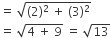SSC CGL Previous Year Questions: Trigonometry - 4 - Question 22

If sin ( A – B ) = 1/2 and cos (A + B) = 1/2 where A > B > 0 and A + B is an acute angle, then the value B is    (SSC CGL 2nd Sit. 2013)

Detailed Solution for SSC CGL Previous Year Questions: Trigonometry - 4 - Question 22

sin (A – B) = 1/2 = sin 30° ⇒ A – B= 30°
cos (A + B) = 1/2 = cos 60° ⇒ A + B = 60°
∴ A + B + A – B = 30° + 60° = 90°
⇒ 2A = 90° ⇒ A = 45°
∴ A – B = 30°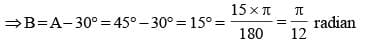SSC CGL Previous Year Questions: Trigonometry - 4 - Question 23

Evaluate : tan 1° tan 2° tan 3° ........... tan 89°.    (SSC CGL 1st Sit. 2013)

Detailed Solution for SSC CGL Previous Year Questions: Trigonometry - 4 - Question 23

tan 89° = tan (90° – 1°) = cot 1°
tan 88° = tan (90° – 2°) = cot 2°
∴ Expression = tan 1°, cot 1°, tan 2°,  cot2° ... tan 45° = 1
[∵ tan θ. cot θ = 1]

SSC CGL Previous Year Questions: Trigonometry - 4 - Question 24

If secθ + tanθ = 2 + √5,  then the value of sinθ + cosθ is?   (SSC CGL 1st Sit. 2013)

Detailed Solution for SSC CGL Previous Year Questions: Trigonometry - 4 - Question 24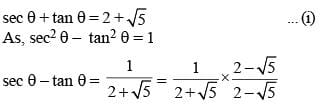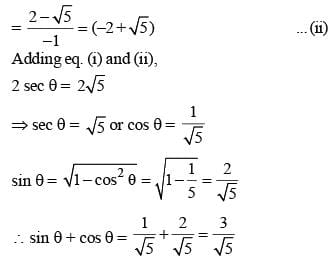SSC CGL Previous Year Questions: Trigonometry - 4 - Question 25

If sinθ + cosecθ = 2, then sin9θ + cosec9θ is equal to ______.    (SSC CGL 1st Sit. 2013)

Detailed Solution for SSC CGL Previous Year Questions: Trigonometry - 4 - Question 25

sinθ + cosecθ = 2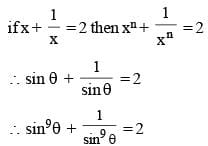SSC CGL Previous Year Questions: Trigonometry - 4 - Question 26

The degree measure of 1 radian (taking π = (22 / 7)) is

Detailed Solution for SSC CGL Previous Year Questions: Trigonometry - 4 - Question 26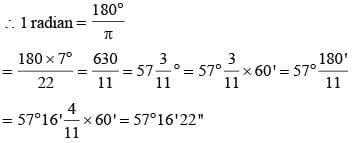SSC CGL Previous Year Questions: Trigonometry - 4 - Question 27

The value of (sin2 25° + sin2 65°) is:    (SSC CGL 1st Sit. 2013)

Detailed Solution for SSC CGL Previous Year Questions: Trigonometry - 4 - Question 27

sin2 25° + sin2 65°
= sin2 25° + sin2 (90° – 25°)
= sin2 25° + cos2 25° = 1

SSC CGL Previous Year Questions: Trigonometry - 4 - Question 28

The value of cos 1° cos 2° cos 3°............ cos 177° cos 178° cos 179° is:    (SSC CGL 1st Sit. 2013)

Detailed Solution for SSC CGL Previous Year Questions: Trigonometry - 4 - Question 28

cos 90° = 0
∴ cos 1°, cos 2° .... cos 179° = 0

SSC CGL Previous Year Questions: Trigonometry - 4 - Question 29

The angle of elevation of a tower from a distance 100 m from its foot is 30°. Height of the tower is:    (SSC CGL 1st Sit. 2013)

Detailed Solution for SSC CGL Previous Year Questions: Trigonometry - 4 - Question 29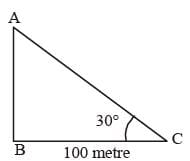AB = h metre
∠ACB = 30°;
BC = 100 metre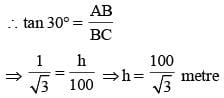SSC CGL Previous Year Questions: Trigonometry - 4 - Question 30

If 2(cos2θ - sin2θ) = 1, (θ is positive acute angle), then cotθ is equal to?   (SSC CHSL 2013)

Detailed Solution for SSC CGL Previous Year Questions: Trigonometry - 4 - Question 30

2 cos 2θ = 1
cos 2θ = 1/2
θ = 30°
cot 30° = √3

SSC CGL Previous Year Questions: Trigonometry - 4 - Question 31

If x sin θ + y cos θ =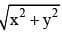and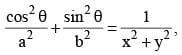then the correct relation is   (SSC CHSL 2013)

Detailed Solution for SSC CGL Previous Year Questions: Trigonometry - 4 - Question 31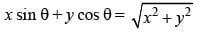Put x = sin θ
y = cos θ in the above equation, we have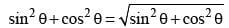⇒ 1 = 1
⇒ x = sin θ & y = cos θ is the solution of above equation. Now, on using x = sin θ & y = cos θ in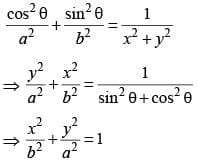SSC CGL Previous Year Questions: Trigonometry - 4 - Question 32

If 5 tan θ = 4, then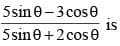(SSC CHSL 2013)

Detailed Solution for SSC CGL Previous Year Questions: Trigonometry - 4 - Question 32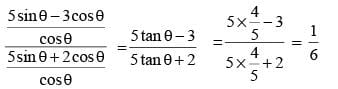SSC CGL Previous Year Questions: Trigonometry - 4 - Question 33

The length of the shadow of a vertical tower on level ground increases by 10 metres when the altitude of the sun changes from 45° to 30°. Then the height of the tower is    (SSC CHSL 2013)

Detailed Solution for SSC CGL Previous Year Questions: Trigonometry - 4 - Question 33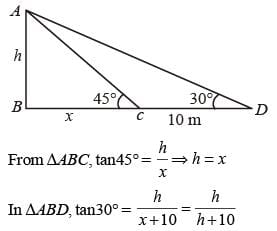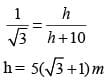SSC CGL Previous Year Questions: Trigonometry - 4 - Question 34

2 cosec2  23° cot 2  67° – sin2  23° – sin2  67°– cot2 67° is equal to    (SSC CHSL 2013)

Detailed Solution for SSC CGL Previous Year Questions: Trigonometry - 4 - Question 34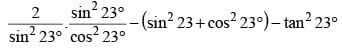= 2 sec2 23° - 1 - tan2 23°
= (sec2 23°- 1) + (sec2 23°- tan2 23°)
= tan2 23° + 1 = sec2 23°

SSC CGL Previous Year Questions: Trigonometry - 4 - Question 35

If cosec θ – cot θ = 7/2, the value of cosec θ is:    (SSC Sub. Ins. 2013)

Detailed Solution for SSC CGL Previous Year Questions: Trigonometry - 4 - Question 35

cosec θ – cot θ = 7/2 ... (i)
cosec2 θ – cot2 θ = 1
⇒ (cosec θ + cot θ) (cosec θ – cot θ) = 1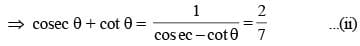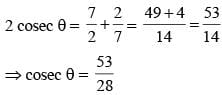SSC CGL Previous Year Questions: Trigonometry - 4 - Question 36

If sin θ – cos θ = 1/2 the value of sin θ + cos θ is:    (SSC Sub. Ins. 2013)

Detailed Solution for SSC CGL Previous Year Questions: Trigonometry - 4 - Question 36

sin θ – cos θ = 1/2
sin θ + cos θ = x.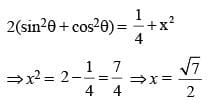SSC CGL Previous Year Questions: Trigonometry - 4 - Question 37

The minimum value of 4 tan2θ + 9 cot2θ is equal to    (SSC Sub. Ins. 2013)

Detailed Solution for SSC CGL Previous Year Questions: Trigonometry - 4 - Question 37

4 tan2 θ + 9 cot2 θ
⇒ (2 tan θ)2 + (3 cot θ)2
(2 tan θ)2 + (3 cot θ)2 – 12 + 12 = (2 tan θ – 3 cot θ)2 + 12
∴ Minimum value = 12 because (2 tan θ – 3 cot θ)2 ≠ 0

SSC CGL Previous Year Questions: Trigonometry - 4 - Question 38

The value of tan 1° tan 2° tan 3° ... tan 89° is:    (SSC Sub. Ins. 2013)

Detailed Solution for SSC CGL Previous Year Questions: Trigonometry - 4 - Question 38

tan 1° tan 2° tan 3° ... tan 89°
= tan 1° tan 2°... tan45° ... tan (90° – 2 ) tan (90° – 1)
= tan 1° tan 2° ... 1 ... cot 2° cot 1°
= (tan 1° cot 1°) (tan 2° cot 2°) ... 1 = 1

SSC CGL Previous Year Questions: Trigonometry - 4 - Question 39

The simplified value of (1 + tan θ + sec θ) (1 + cot θ – cosec θ) is    (SSC CGL 2nd Sit. 2012)

Detailed Solution for SSC CGL Previous Year Questions: Trigonometry - 4 - Question 39

(1 + tan θ + sec θ) (1 + cot θ – cosec θ)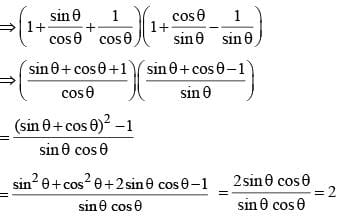SSC CGL Previous Year Questions: Trigonometry - 4 - Question 40

If sin2α = cos3α, then the value of (cot6α – cot2α) is    (SSC CGL 2nd Sit. 2012)

Detailed Solution for SSC CGL Previous Year Questions: Trigonometry - 4 - Question 40

If sin2α = cos3α
tan2α = cosα ...(1)
Now consider, cot6α – cot2α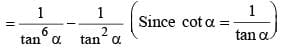Substituting for tan2α with cos α from (1) above equation will be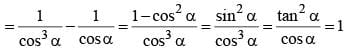## SSC CGL (Tier - 1) - Previous Year Papers (Topic Wise)

250 tests
Information about SSC CGL Previous Year Questions: Trigonometry - 4 Page
In this test you can find the Exam questions for SSC CGL Previous Year Questions: Trigonometry - 4 solved & explained in the simplest way possible. Besides giving Questions and answers for SSC CGL Previous Year Questions: Trigonometry - 4, EduRev gives you an ample number of Online tests for practice

250 tests